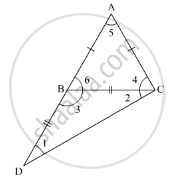Advertisement Remove all ads

# In a Triangle Abc, If Ab = Ac and Ab is Produced to D Such that Bd = Bc, Find ∠Acd: ∠Adc. - Mathematics

Answer in Brief

In a triangle ABC, if AB =  AC and AB is produced to D such that BD =  BC, find ∠ACD: ∠ADC.

Advertisement Remove all ads

#### Solution

In the given ΔABC,AB = ACand AB is produced to D such that  BD = BC

We need to find  ∠ACD : ∠ADCNow, using the property, “angles opposite to equal sides are equal”

As  AB = AC

∠6 = ∠4          ........(1)

Similarly,

As   BD = BC

∠1 = ∠2              ........(2)

Also, using the property, “an exterior angle of the triangle is equal to the sum of the two opposite interior angle”

In ΔBDC

ext. ∠6 = ∠1 + ∠2

ext. ∠6 = ∠1 + ∠1 (Using 2)

ext. ∠6 = 2∠1

From (1), we get

ext. ∠4 = ∠2        .......(3)

Now, we need to find  ∠ACD : ∠ADC

That is,

(∠4 + ∠2): ∠1

(2∠1 + ∠2) : ∠1 (Using 3)

(2∠1 + ∠1) : ∠1(Using 2)

3∠1 :∠1

Eliminating ∠1from both the sides, we get 3:1

Thus, the ratio of ∠ACD :∠ADC is 3 :1

Is there an error in this question or solution?
Advertisement Remove all ads

#### APPEARS IN

RD Sharma Mathematics for Class 9
Chapter 11 Triangle and its Angles
Q 12 | Page 24
Advertisement Remove all ads

#### Video TutorialsVIEW ALL 

Advertisement Remove all ads
Share
Notifications

View all notifications

Forgot password?
Course